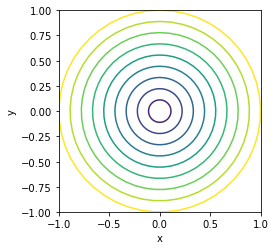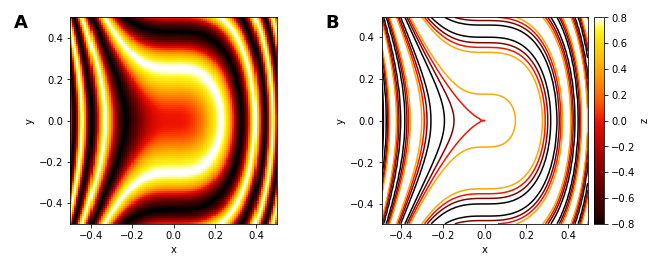# Contours¶

Title
Contours Element
Dependencies
Matplotlib
Backends
Matplotlib
Bokeh
In :
import numpy as np
import holoviews as hv
hv.extension('matplotlib')A Contours object is similar to a Path element but allows each individual path to be associated with one or more scalar values declared as value dimensions (vdims), which can be used to apply colormapping the Contours. Just like the Path element Contours will accept a list of arrays, dataframes, a dictionaries of columns (or any of the other literal formats including tuples of columns and lists of tuples). In order to efficiently represent the scalar values associated with each path the dictionary format is preferable since it can store the scalar values without expanding them into a whole column. For a full description of the path geometry data model see the Geometry Data User Guide.

To see the effect we will create a number of concentric rings with increasing radii and define a colormap to apply color the circles:

In :
def circle(radius):
angles = np.linspace(0, 2*np.pi, 50)
return {'x': radius*np.sin(angles), 'y': radius*np.cos(angles), 'radius': radius}

hv.Contours([circle(i) for i in np.linspace(0, 1, 10)], vdims='radius')

Out:Often Contours will be directly computed from an underlying Image, which is made easy using the contours operation. The operation accepts an Image type as input and will return Contours containing iso-contours for each of the specified levels. We will declare an Image of sine rings and then compute Contours at 5 levels spaced linearly over the range of values in the Image:

In :
x,y = np.mgrid[-50:51, -50:51] * 0.05
img = hv.Image(np.sin(x**2+y**3))
contours = hv.operation.contours(img, levels=5)

img + contours.opts(colorbar=True, cmap='fire')

Out:For full documentation and the available style and plot options, use hv.help(hv.Contours).

Download this notebook from GitHub (right-click to download).